# AP Physics C Electricity : Using Ohm's Law

## Example Questions

### Example Question #1 : Circuit Relationships

A simple circuit consists of a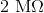resistor that's connected to a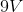battery. How much power is dissipated by the resistor?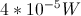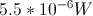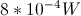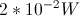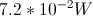Explanation:

One of the formulas for power is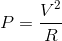. We are given the values of the voltage and resistance.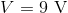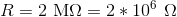Using these values, we can solve for power.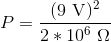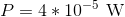### Example Question #1 : Circuit Relationships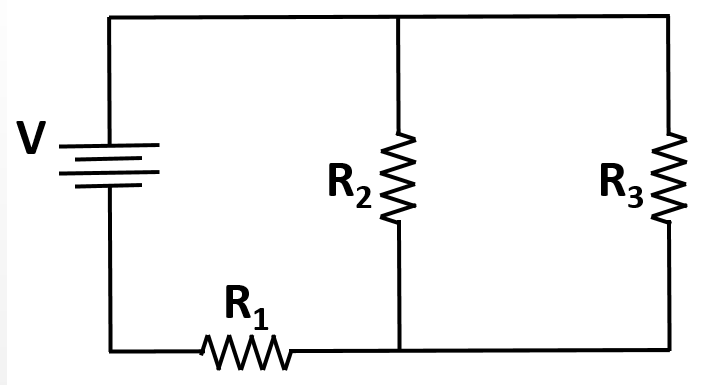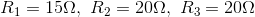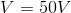What is the current delivered to the curcuit by the battery?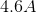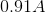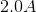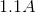Explanation:

Using Ohm's law to solve for the value of the current from the battery requires calculation of the equivalent resistance of the circuit.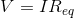The resistors R2 and R3 are in parallel with one another. Once combined, their requivalent resistor (R23) is in series with R1.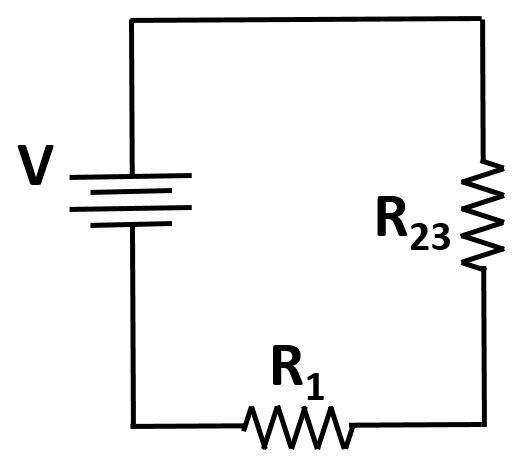The equivalent resistance is thus given by: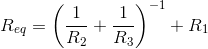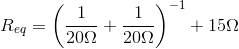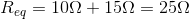Use this value and the given voltage to solve for the current in the circuit: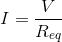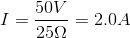### All AP Physics C Electricity Resources